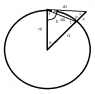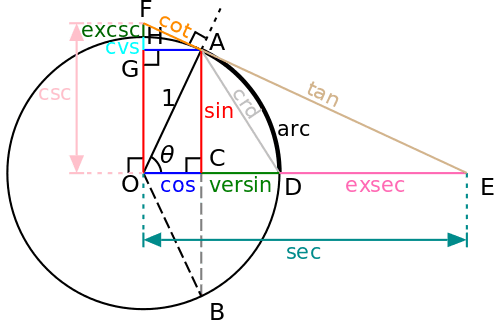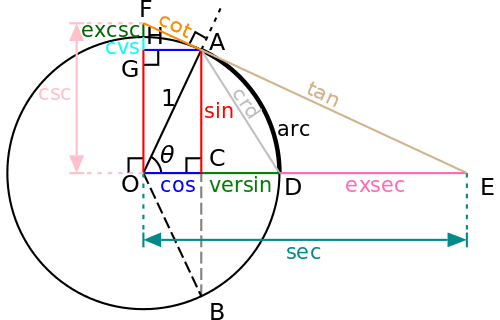# Finding a new way to calculate one side of a triangle

Gold Member
hopefully this is the right place for this question, the first part is a trig/geometry question but it is really a integration question:

i'm trying to find another way to compute d1 without using law of cosines because i don't know how to integrate (cos a)^.5, if someone knows how to do that please let me know.

in the figure provided I'm attempting to find d1 as a function of angle a, r0 and r1 are constants and angles t, p and lengths d0, x can be computed trivially.

i can use law of sines to find d1 if i can make b, m or c a function of a

#### Attachments

•distance function.png
2.2 KB · Views: 447
Last edited:

Homework Helper
...r0 and r1 are constants...
If r1=r0+x, then d1 is related to r0 and r1 by pythagoras.

Staff Emeritus
Homework Helper
This diagram is a handy way to visualize the relationship between the trig functions and the angle using a unit circle: (credit: wikipedia)Gold Member
If r1=r0+x, then d1 is related to r0 and r1 by pythagoras.

yes, r1=r0+x, but there are no right angles in the figure

Gold Member
This diagram is a handy way to visualize the relationship between the trig functions and the angle using a unit circle: (credit: wikipedia)thanks i'll reflect on this, but i suspect that this would only be useful in the case where i have right angles

Homework Helper
yes, r1=r0+x, but there are no right angles in the figure
Ah - so d1 is not a tangent ... it looks a lot like it is supposed to be one in the figure.

This is where I suspect you need to look for another way to set up the integral - or learn to use elliptic integrals.

Gold Member
Ah - so d1 is not a tangent ... it looks a lot like it is supposed to be one in the figure.

This is where I suspect you need to look for another way to set up the integral - or learn to use elliptic integrals.

can you give me some good lead for elliptic integrals? thansk

Gold Member
$$\int \cos^{\frac{1}{2}}\!(x)\; dx = 2E\big(\frac{x}{2}\big|2\big)$$

##E(x|m)##
Elliptic Integral of the seconds kind [properties]

thanks for your help, but I've looked through some basic elliptical integrals and I'm not finding anything like (cos x)^.5 the basic form is 1-(sin x )^2

also what I'm working with is not an ellipse it is a circle. the difference is that instead of a constant radius from the center tracing out the circle it is circle traced from a point off center and instead of a constant radius length the this segment length changes

Homework Helper
"elliptic integral" not "elliptical".
It is the name for a class of functions - they have application beyond elliptic curves.

http://integrals.wolfram.com/index.jsp?expr=sqrt(cos(x))&random=false

Um - note: if x is very small compared with r0, then cos(m)~1 and sin(m)~m and tan(m)~m.

In the diagram in post #3 - your triangles are AOD and ADE.
The exsec side is you side x.
AO=OD=r0
... like that.

Last edited:
•1 person
Gold Member
thanks to simon I've figured out the simple elliptic however i now have a more difficult version:

$$\int_0^{2 \pi } \sqrt{\left(1-\frac{60}{61} \cos (x-179)\right) \left(1-\frac{60}{61} \cos (x-t)\right)} \, dx$$

using mathematica i can find the solution to

$$\int \sqrt{1-\frac{60}{61} \cos (x-t)} \, dx$$

but mathematica was not able to solve the harder version, any ideas?

also how do get the latex to show correctly?

thanks mark44

Last edited:
Mentor
thanks to simon I've figured out the simple elliptic however i know have a more difficult version:

\int_0^{2 \pi } \sqrt{\left(1-\frac{60}{61} \cos (x-179)\right) \left(1-\frac{60}{61} \cos (x-t)\right)} \, dx

using mathematica i can find the solution to

\int \sqrt{1-\frac{60}{61} \cos (x-t)} \, dx

but mathematica was not able to solve the harder version, any ideas?

also how do get the latex to show correctly?
Add $$before and after your LaTeX. Here's what your stuff looks like with this addition.$$\int_0^{2 \pi } \sqrt{\left(1-\frac{60}{61} \cos (x-179)\right) \left(1-\frac{60}{61} \cos (x-t)\right)} \, dx \int \sqrt{1-\frac{60}{61} \cos (x-t)} \, dx
$$Science Advisor Homework Helper$$\left(1-\frac{60}{61} \cos (x-179)\right) \left(1-\frac{60}{61} \cos (x-t)\right)$$... hmmm? expand the brackets and try trig identities? A quick path can be to expand the trig function by the Euler relations since manipulating exponentials is usually easier. Gold Member thanks simon, are you referring to$$e^{\text{ix}}=x \cos + i x \sin